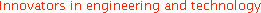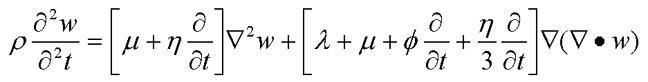Wave2000®; is a stand-alone computer software program for computational ultrasonics. It was produced to bring numerical simulation to the ultrasound engineering community. This page provides some basic information about Wave2000® including a general Program Overview, Hardware/Software/Memory Requirements, and a brief description of the Wave Equation that is being simulated.

Program Overview

Wave2000® is a stand-alone software package for computational ultrasonics. It operates by solving the two-dimensional (2D) acoustic (elastic and visco-elastic) wave equation based on a method of finite differences. The solution is computationally intensive. However, thanks to the ever increasing power and speed of computer hardware, we at CyberLogic felt the time was right to bring such software packages to the desktop and personal computer of the ultrasound engineer and researcher.

Wave2000® allows the user to compute the full acoustic wave solution in an arbitrary two-dimensional (2D) object, subjected to user-specified acoustic sources. The program, besides simulating the complete spatial and time-dependent acoustic solution, allow the user to simulate ultrasound measurements in a variety of source and receiver configurations. Wave2000® will prove useful to researchers and applications engineers in such diverse fields as non-destructive testing, materials evaluation, medical imaging and biological tissue characterization. The software is useful also in basic mechanistic studies and for academic applications. With Wave2000® the user can compute solutions (e.g., scattered and reflected waveforms) to virtually an unlimited variety of distinct physical problems without ever leaving the computer keyboard.

Wave2000® provides solutions to a broad range of 2D ultrasound problems. The program allows the user to specify an arbitrary object which is ultrasonically interrogated. The object is specified in a "PCX" image file. "PCX" is a "tried-and true" graphics file format which includes within it absolute size information, a useful feature for the ultrasound simulations. The image data or object is composed of individual pixels which can have 1 of 256 gray levels (0-255). Each pixel value represents a physical material (e.g., water, steel, etc.) that is set by the user. Gray level 255 is reserved by Wave2000® to denote void (i.e., vacuum). There is thus a vast variety of 2D structures which can be processed using Wave2000®.

The 2D object or image can be generated either internally using Wave2000® "Geometry" routines or externally using any graphics program (commercial or "in-house") which can output files in 8 bit monochrome "PCX" format. Additionally, the image may be obtained from various scan modalities, for example CT or MRI slice data, which has been converted to "PCX" format. Usually, a segmentation algorithm of some kind would be necessary to properly associate various regions of the image with a particular material (i.e., grey level). Although Wave2000® computes solutions to 2D ultrasound problems, the solutions can be viewed as applying to three-dimensional objects which happen to be "quite long" in the dimensions going into and out of the object plane. Of course, by "quite long" we mean that the object extends from minus infinity to plus infinity, and that there also is no change in the object cross-section along this dimension. Of course this is hardly a "practical" experiment; nevertheless, the 2D simulations take on a bit more "real world feel" when viewed in this "3D" way.

The attractiveness of Wave2000® is that it is very easy to generate solutions to a wide variety of 2D ultrasound problems within a simple graphical interface. The user has access to features designed to mimic reasonably closely many practical situations. For example, there are source and receiver configurations that the ultrasound engineer will notice are similar to real ultrasound experimental configurations, for example the use of signals that characterize many transducer generated waveforms. As one application, Wave2000® may be useful for analyzing 2D wave propagation associated with transducer-like structures (e.g., layered) subject to various temporal (source) and static (boundary condition) displacement boundary conditions, in the design of ultrasound transducers.

Wave2000® has the potential to generate new insights and approaches to many problems in ultrasonics. For the first time, the user has the capability to "experiment" to his or her heart's content, without turning on a pulser-receiver or connecting a "BNC" cable. Indeed, the simulations can "run" while the user is word processing a document or analyzing data from an earlier simulation. The computer can work "around the clock" computing solutions to problems that are difficult to perform in the laboratory or the field, but the results of the simulations can provide important understanding and knowledge for future experiments or for data already collected.

##### Back to the top

Hardware, Software and Memory Requirements

Wave2000® is designed to run on any PC that has the Windows XP, Vista, 7 or 8 operating systems installed. Minimum hardware requirements are extremely modest (for example 15 megabytes free hard disk space and 128 megabytes memory), although we recommend 1 GB or more memory for handling the larger simulation models. (Detailed information on memory requirements can be found in the 'Algorithm' topic of the Wave2000® User Guide Section of the Help file.) Minimum graphics requirements are 256 color VGA and a compatible mouse, but of course most systems will have hardware characteristics much higher than these minimums. As noted, Wave2000® operates best with as much RAM memory as possible, which allows problems of increasingly larger size to be accommodated and avoids the need for virtual (disk) memory to be used. Note also that Wave2000® supports multiprocessor systems, that allows the program to potentially speed-up the execution of the program. This is most effective for "large" objects; experimentation by the user will determine when multiprocessors can lead to faster execution times. The multiprocessors can be either actual multiproccessor systems, or multicore processors, or both.

Wave2000® is a "memory-hungry" program; this is simply the nature of the acoustic problem which is being simulated. To aid the user in assessing the memory requirements for a particular simulation model, we can provide the following approximate relationships.

The simplest approach for approximating memory needed is to multiply the number of finite difference grid elements, N, by 30; this is the amount of memory required in bytes (plus some additional "fixed" program overhead which can usually be neglected in comparison to the 30 x N quantity). As an example, if an object image is 3 cm x 4 cm and the pixel resolution ("Pixels/mm") is 10 pixels/mm, the number of pixels in this image will be 300 x 400 = 120,000 pixels. Now if we assume that the finite difference grid elements generated by Wave2000® are coincident with the number of image pixels (i.e., Grid/Pixel = 1), then the memory required is 30 x N = 30 x 120,000 = 3.4 megabytes.

Another perspective on memory requirements can be gained by determining the memory needed as a function of object size in terms of wavelengths. In the example above, it was assumed the the grid size was coincident with object image pixel size, i.e., both were 0.1 mm square (10 pixels/mm). For the case of a problem in which the minimum wavelength is about 1 mm, this 0.1 mm size for the finite difference grid element should provide a reasonably accurate solution. We may extend this reasoning for a more generic assessment of memory requirements as follows. Assuming that we would like to have a grid element dimension 10 times less than the minimum wavelength, then that implies that 100 x 30 = 3000 bytes for a square object 1 wavelength on a side. If one has a square object which is 10 wavelengths on a side, then using the same relative grid dimensions, Wave2000 would require about 10,000 x 30 = 293 kilobytes of memory. One may also extend this approximation to any number of (minimum) wavelengths to evaluate memory requirements. Assuming a rectangular object Q wavelengths by R wavelengths in overall dimensions, and again assuming 10 grid elements per wavelength, then the memory required for this model is approximately 30 x 100 x Q x R = 2.93 Q x R kilobytes. It is useful to also point out that the minimum wavelength is inversely proportional to the frequency of the source waveform. Therefore, if a simulation with a 1 MHz source waveform requires 1 megabyte of memory, then changing to a source waveform operating at 2 MHz will generally require four times as much memory, or in this case 4 megabytes, to be used. (This assumes that the same size object is used in both cases.) Thus the user may want to carry out as many of his or her simulations as possible with relatively low frequency sources, in order to reduce computational overhead.

##### Back to the top

Wave Equation

The specific acoustic equation that is simulated in a Wave2000® simulation is given by:In the above equation, which applies in an isotropic elastic region,

ρ = material density [kg/m^3],

λ = first Lame constant [N/m^2],

μ = second Lame constant [N/m^2],

η = shear viscosity [N-s/m^2],

φ = bulk viscosity [N-s/m^2],

∇ • = the divergence operator,

denotes the partial differential operator,

t = time [s],

and

w is a two dimensional column vector whose components are the x and y components of displacement of the medium at location (x,y), that is

w = [wx(x,y,t) wy(x,y,t)]'

where ' denotes matrix transpose. Wave2000 solves the above equation within each homogenous grid element of the object, and computes (and displays) the displacement vector at the intersection of 4 grid elements at each time step of the simulation. As noted above, the simulation explicitly satisfies the stress and displacement boundary conditions across each and every grid element of the simulation model. Additional information on the acoustic wave equation including the viscous loss component (i.e., the viscosity tensor) can be found in the excellent book by B. A. Auld entitled Acoustic Fields and Waves in Solids, Vols. 1-2, 2nd Edition, Krieger Publishing Company, Malabar, Florida, 1990.

Wave2000® does not implement "ray-tracing" or other "non-general" methods in simulating ultrasound measurements. Rather, it is a comprehensive engineering software package designed to compute the full and accurate solution to practically any 2D ultrasonic problem. Wave2000® simulates data that you would measure on the lab bench or in the field. In addition, it has an easy to use graphical user interface allowing you to begin simulating complex ultrasound problems in a matter of minutes after receiving your Wave2000® software.

Back to the top